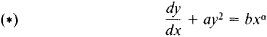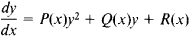# Riccati Equation

(redirected from Riccati differential equation)
Also found in: Acronyms.

## Riccati equation

[ri′käd·ē i‚kwā·zhən]
(mathematics)
A first-order differential equation having the form y ′ = A0(x) + A1(x) y + A2(x) y 2; every second-order linear differential equation can be transformed into an equation of this form.
A matrix equation of the form dP (t)/ dt + P (t) F (t) + F T (t) P (t) -P (t) G (t) R -1(t) G T (t) P (t) + Q (t) = 0, which frequently arises in control and estimation theory.
McGraw-Hill Dictionary of Scientific & Technical Terms, 6E, Copyright © 2003 by The McGraw-Hill Companies, Inc.
The following article is from The Great Soviet Encyclopedia (1979). It might be outdated or ideologically biased.

## Riccati Equation

a first-order ordinary differential equation of the formwhere a, b, and a are constants. This equation was first investigated by J. Riccati in 1724; certain special cases were studied earlier. In work done in 1724 and 1725, D. Bernoulli established that equation (*) can be integrated in terms of elementary functions when α = – 2 or α = – 4k/(2k – 1), where k is an integer. J. Liouville proved in 1841 that, for other values of α, the equation (*) cannot be solved by quadrature, that is, by applying a finite number of algebraic operations, transformations of variables, and indefinite integrations to elementary functions; a general solution can be expressed in terms of cylindrical functions.

The differential equationwhere P(x), Q(x), and R(x) are continuous functions, is called the generalized Riccati equation. When P(x) = 0, the generalized Riccati equation is a linear differential equation; when R(x) = 0, it is the Bernoulli equation. In these two cases, the equation is integrable in closed form. Other cases of the integrability of the generalized Riccati equation have also been studied.

### REFERENCE

Kamke, E. Spravochnik po obyknovennym differentsial’nym uravneniiam, 4th ed. Moscow, 1971. (Translated from German.)
Mentioned in ?
References in periodicals archive ?
where P [greater than or equal to] 0 [member of] [R.sup.mxm] satisfies Riccati differential equation (24).
In this section, we use the generalized Legendre wavelet operational matrix to solve nonlinear Riccati differential equation and we discuss the existence and uniqueness of solutions with initial conditions and convergence criteria of the proposed LWM approach.
Consider the fractional-order Riccati differential equation of the form
One can see that CWLM is accurate and is able to solve this nonlinear Riccati differential equation in a very wider region.
To overcome the limitations and shortcoming of the existing methods, the new multiagent NN approach is proposed to solve nonlinear fuzzy Riccati differential equation.
The main objective in this paper is to introduce two efficient spectral algorithms based on using ultraspherical wavelets for solving fractional-order Riccati differential equation. The two algorithms, namely, ultraspherical wavelets tau method (UWTM) and ultraspherical wavelets collocation method (UWCM), are implemented and presented for handling both of linear and nonlinear fractional-order Riccati differential equations.
In this study, we present a Taylor collocation method for the numerical solutions of fractional Riccati differential equation with delay term.
Zhang, "A generalized exp-function method for fractional Riccati differential equations," Communications in Fractional Calculus, vol.
LAUB, Efficient matrix-valued algorithms for solving stiff Riccati differential equations, IEEE Trans.
Hosseini Matikolai, "Homotopy perturbation pade technique for solving fractional Riccati differential equations," International Journal of Nonlinear Sciences and Numerical Simulation, vol.
Hosseini Matikolai, "Homotopy perturbation pade technique for solving fractional Riccati differential equations," The International Journal of Nonlinear Sciences and Numerical Simulation, vol.

Site: Follow: Share:
Open / Close